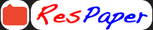Trending ▼   ResFinder# ICSE Class X Prelims 2021 : Mathematics (Lady Ratanbai and Sir Mathuradas Vissanji Academy, Mumbai)

4 pages, 44 questions, 1 questions with responses, 0 total responses,20Rishi Baria +Fave Message ProfileTimelineUploads
 Home > rishibaria >   F Also featured on: School Page icse and 2 moreFormatting page ...

L.R. & S.M. Vissanji Academy Secondary Section Second Priliminary Examination 2021 SUBJECT : Mathematics Grade : 10 Date : 22 / 03 /21 Marks : 80 Time: hrs INSTRUCTIONS: Answers to this paper must be written on the paper provided separately. You will not be allowed to write during the first 15 minutes. This time is to be spent in reading the question paper. The time given at the head of this paper is the time allowed for writing the answers. This paper has_5_ printed pages. The intended marks for the questions or parts of questions are given alongside the questions. Section I  Attempt all questions in this section. Question 1 1 i) Find 5 numbers in AP, whose sum is 12 and ratio of first to last term is 2:3.  2 4 1 ii) Given M = [ ]. Find k if M2 6M + kI = Null Matrix,  1 2 where I is an identity matrix of order 2 x 2 . iii)There are two dice, one red and the other black. Both are rolled.  Simultaneously .Calculate the probability that (a) the number on the red dice is 3. (b) each dice shows 5 (c) the number on the black dice is either 2 or 4 (d) the product of two numbers is odd. Question 2 i) Solve 2 +1 2 + 2(3 ) 7, . Also graph the solution set on the number line. i) solve the quadratic equation x2 -3(x+ 3) = 0; give your answer correct to two significant figures.  iii) If 2x3 + ax2 + bx- 2 has a factor ( x+ 2) and leaves a remainder 7 when divided by 2x -3 , find the value of a and b.with these values of a and b, factorise the given polynomial completely.Formatting page ...Formatting page ...Formatting page ...© 2010 - 2021 ResPaper. Terms of ServiceContact Us Advertise with us

ICSE Q&A - Ask and Answerrishibaria chat